Courses

# Second Law Of Thermodynamic MCQ Level – 1 (Part - 2)

## 10 Questions MCQ Test Topic wise Tests for IIT JAM Physics | Second Law Of Thermodynamic MCQ Level – 1 (Part - 2)

Description
This mock test of Second Law Of Thermodynamic MCQ Level – 1 (Part - 2) for IIT JAM helps you for every IIT JAM entrance exam. This contains 10 Multiple Choice Questions for IIT JAM Second Law Of Thermodynamic MCQ Level – 1 (Part - 2) (mcq) to study with solutions a complete question bank. The solved questions answers in this Second Law Of Thermodynamic MCQ Level – 1 (Part - 2) quiz give you a good mix of easy questions and tough questions. IIT JAM students definitely take this Second Law Of Thermodynamic MCQ Level – 1 (Part - 2) exercise for a better result in the exam. You can find other Second Law Of Thermodynamic MCQ Level – 1 (Part - 2) extra questions, long questions & short questions for IIT JAM on EduRev as well by searching above.
QUESTION: 1

### T-S diagram for Carnot cycle is. Select one:

Solution: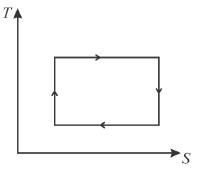QUESTION: 2

### For the thermodynamic process shown in the figure, corresponding T-S diagram is.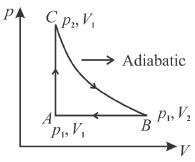Select one:

Solution:

Process C to B is adiabatic
it is is isentropic process.
Hence, (b) and (d) cannot be correct.
B to A is isobaric process but in option (c), B to A is isothermal.
Hence (c) is also not correct.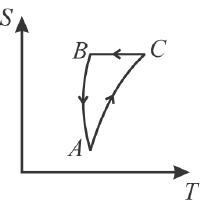QUESTION: 3

### All natural processes are irreversible. This is a direct consequence of. Select one:

Solution:

An irreversible processes increases the entropy of the universe. However, because entropy is a state function, the change in entropy of the system is same whether the process is reversible or irreversible.
All the natural processes are irreversible. This irreversibility results from the fact that if a thermodynamic system, which is any system of  sufficient complexity, of interacting molecules is brought from one thermodynamic state to another, the configuration or arrangement of the atoms and molecules in the system will change in a which that is not easily predictable. During this transformation, there will be a certain amount of heat energy loss or dissipation due to intermolecular friction and collision, energy  that will not be recoverable if the process is reversed.
The correct answer is: Second law of thermodynamics

QUESTION: 4

In a refrigerator, the heat exhausted to the outer atmosphere is.
Select one:

Solution: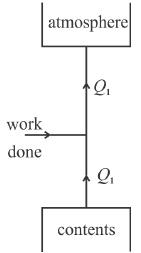Q1 = W + Q2
Hence   Q1 > Q2.
The correct answer is: more than that absorbed from the contents

QUESTION: 5

A given amount of heat cannot be completely converted into work. However, it is possible to convert a given amount of work completely in heat. This statement results from the
Select one:

Solution:

The above statement comes from Kelvin-Plank’s statement of second law of thermodynamics.
The correct answer is: Second law of thermodynamics

QUESTION: 6

The temperature of the surface of the sun is approximately 6000K. It we take a big lens and focus the sun rays and produce a temperature of 8000K. This will violate
Select one:

Solution:

The creation of a temperature of 8000K by transferring heat from a colder body (sun at 6000K) by means of a lens is a violation of second law of thermodynamics.
The correct answer is: Second law

QUESTION: 7

The area of the Carnot cycle on a T-S diagram represents
Select one:

Solution:

The total area under top side of rectangle is total heat absorbed. The area under bottom side is total heat released. The area inside the rectangle is total heat absorbed- total heat released = total work done in a cycle.”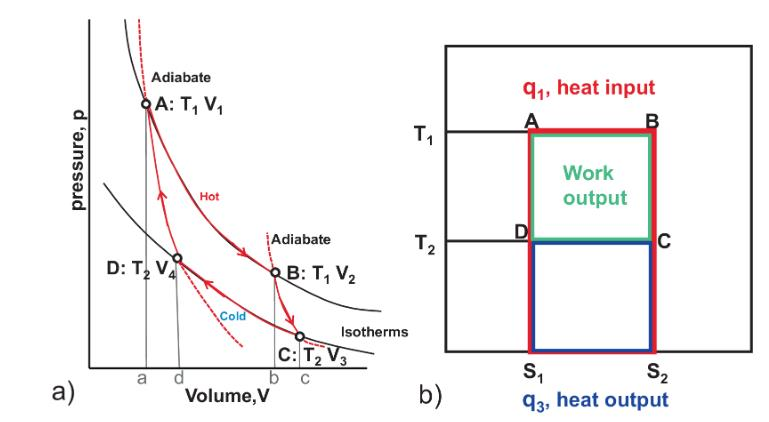QUESTION: 8

For any process, the second law of thermodynamics requires that the change of entropy of universe is.
Select one:

Solution:

From second law thermodynamics,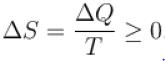The correct answer is: positive or zero

QUESTION: 9

For a reversible cyclic process, the value of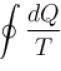is.
Select one:

Solution:

For a reversible cycle process just like the Carnot engine,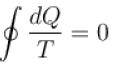This is because any reversible cycle can be represented as a series of miniature Carnot cycle.
The correct answer is: always equal to zero

QUESTION: 10

In a heat engine maximum heat that can be converted into mechanical work.
Select one:

Solution:

Since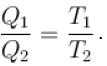The work temperature plays an important role in the efficiency of the engine.
The correct answer is: depends upon working temperature